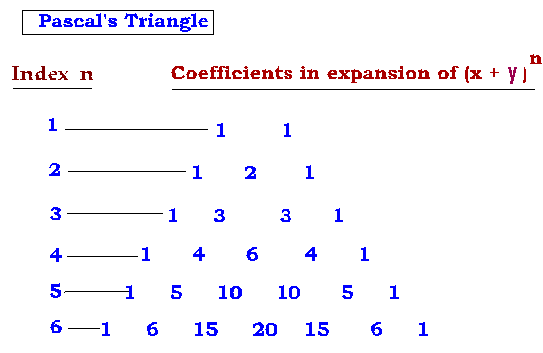## 1. Binomial expression, binomial expansion, binomial theorem

Any expression that contains two terms is called a binomial expansion.
For eg: 2x + 3y, (x2 – 1/x2 )

## 2. Binomial Index

The power to which the binomial is raised is called binomial index.
For eg in (3 – 2y )10, the index (binomial index) is 10.

## 3. Binomial coefficients

In the binomial expansion
(x+y)n = nc0 xn + nc1 xn-1 y + nc2 xn-2 y2 + nc3.xn-3 y3 +……. + ncr.xn-r yr +…. + ncn.yn
nc0 , nc1 , nc2 , ……….ncr , ………..ncn

are called binomial coefficients

## 4. Pascal’s triangle.## 5. Factorial of a number n, n!

The product of all numbers from 1 upto n is called factorial of number n
It is denoted as n!
n! = n (n – 1 ) ( n – 2 ) (n – 3 )(n – 4 ) ………3.2.1
for eg, 6! = 6.5.4.3.2.1 = 720

## 6. General term

In the binomial expansion:
(x+y)n = nc0 xn + nc1 xn-1 y + nc2 xn-2 y2 + nc3.xn-3 y3 +……. + ncr.xn-r yr +…. + ncn.yn
ncr.xn-r . yr
is called the general term. To find any term in any binomial expansion, the general term is used.

## 7. Middle term

In any binomial expansion, a particular term is equidistant from the first term and the last term. This is called the middle term.
Only one middle term is there in a binomial expansion whose binomial index n is an even integer,and two middle terms if the binomial index n is an odd integer.

## 8. Independent term

Sometimes, in some binomial expansions, there will be a term having no x. This is because the power of x in the term reduces to 0 and so x vanishes to become 1. Such a term which does not contain x is called Term Independent of x

## 9. Greatest binomial coefficient

As the terms in a binomial expression are written, the binomial coefficients steadily increase from 1, reach a peak, and start again to decrease to 1.
The binomial coefficient which is greatest in value is called the greatest binomial coefficient. Greatest binomial coefficient depends upon binomial index n.
If binomial index n is even integer, there is only one greatest binomial coefficient.
If binomial index n is an odd integer, there are two greatest but equal binomial coefficients.

## 10. Numerically greatest term

As the terms of a binomial expression are written, there will be a term or terms containing the greatest number. This term is called the numerically greatest term.
A particular term in the binomial expansion will have the greatest number due to the product of the binomial coefficients and the coefficients of the variables in the binomial expression. It is called numerically greatest because, the number resulting from the product of the coefficients can have a negative sign. But the negative sign is disregarded and discarded only to find the greatest number among all that are available in all of the terms.

## 11. Numerical value

Numerical value refers to only the numeral in a number without regard for the sign. For eg,numbers 2 and -2 are different. But their numerical values are same, which is just 2.

## 12. Even and Odd binomial coefficients:

In the binomial expansion :
(x+y)n = nc0 xn + nc1 xn-1 y + nc2 xn-2 y2 + nc3.xn-3 y3 +……. + ncr. xn-r yr +…. + ncn.yn
among the binomial coefficients:
nc0 , nc1 , nc2 , ……….ncr ,………..ncn
a few are called even binomial coefficients and the others, odd binomial coefficients.
The binomial coefficients are called odd or even binomial coefficients depending on the value of r.
If r is even, then the binomial coefficients are called even binomial coefficients.
Eg: nc0 , nc2 , nc4 , are called even binomial coefficients. And
nc1 , nc3 , nc5 are called odd binomial coefficients.

Comment Box is loading comments...